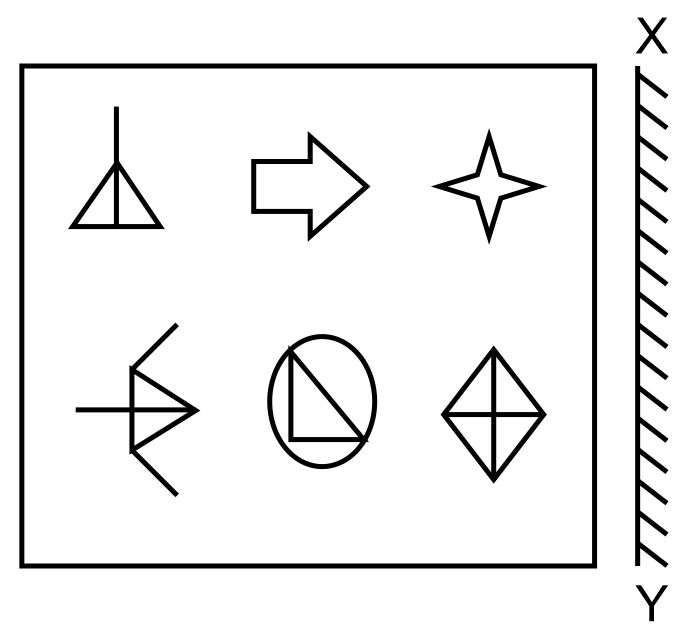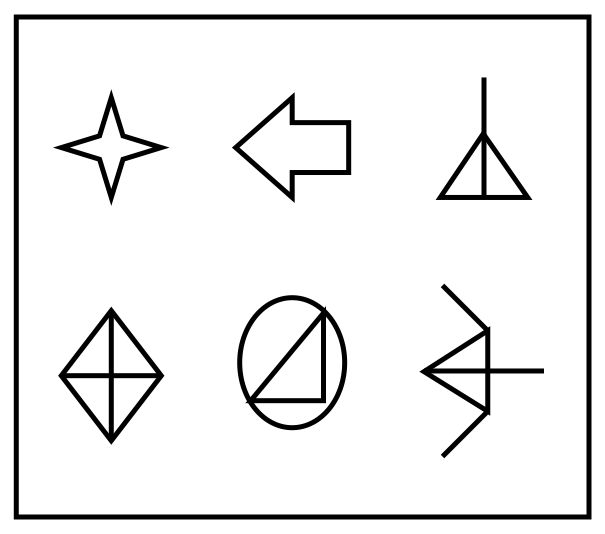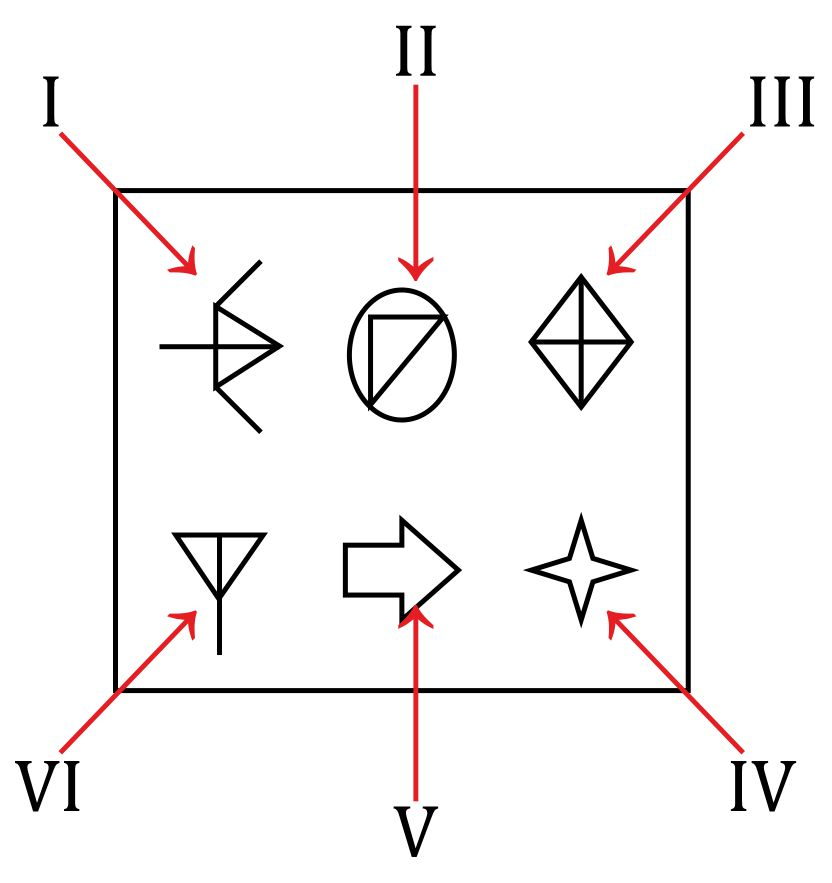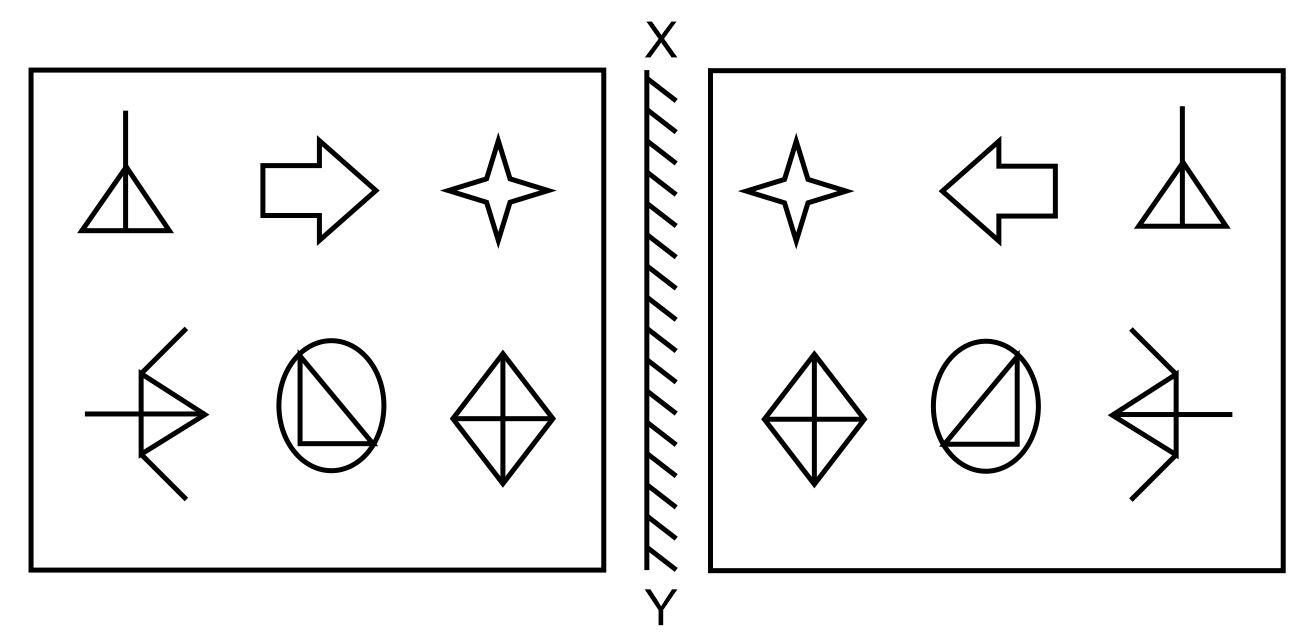Question of The Day27-11-2019

Which of the following options shows the mirror image of the question about the XY axis?Correct Answer : d )Explanation :

To find the mirror image of any object, we have to consider the following rules/points:

• The Left-hand side portion of the image appears on the right side in the mirror image while the right hand side portion of the image appears on the left-hand side in the mirror image.
• The portion of the image, which is bilaterally symmetrical will appear the same in the mirror image.

Considering the given imageThe mirror image of the above image will follow the below points:

1. In the above image, figure III, figure IV and figure VI will be bilaterally symmetrical about the vertical axis passing through their centers so these images will appear the same in the mirror image.
2. In figure I, the bulged right half part will appear on the left side in the mirror image.
3. In figure V, the arrow sign will appear pointing towards the left side in the mirror image.
4. Figures I, II, and III will appear in the upper half of the mirror image.
5. Figures IV, V, and VI will appear in the lower half of the mirror image.

Now, considering option (A): In this option point 4 and 5 are not satisfied thus option (A) fails

Now, considering option (B): In this option, point (1) is not satisfied thus, option (B) fails.

Now, considering option (C):  In this option, point (2) is not satisfied thus, option (C) also fails.

Considering option (D): Considering all the rules and points we can say that

The mirror image of the question figure will be:Hence, (D) is the correct answer.

Such type of question is asked in various government exams like SSC CGL, SSC MTS, SSC CPO, SSC CHSL, RRB JE, RRB NTPC, RRB GROUP D, etc. Try and attempt free mock tests at PendulumEdu.

Ready Daily Current Affairs to boost your preparation for the General Awareness section.0